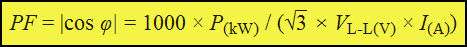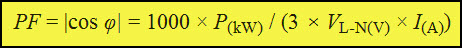# Power Factor Calculation

Power factor is the significant factor for evaluating the effectiveness of usage of electric power in a power system network. If the power factor is good or high (unity), then we can say that more effectively the electric power is being used in a power system. As the power factor is poor or reduces, the effectiveness of usage of electric power reduces in power system. The poor power factor or power factor reduction is caused by various reasons. So, for improving power factor there are various power factor correction techniques. The power factor correction using power factor correction capacitors is the best and efficient method of various power factor correction methods. But, primarily we must know what is power factor, power factor calculation, and power factor correction.

### What is Power Factor?

A power factor can be described in various terms such, it can be called as the ratio between active power and apparent power, it can be defined as the cosine of angle between voltage and current. The cosine of the angle between voltage and current is considered (not sine or tangent or cotangent angle), because the phasor diagram of voltage or current from power triangle is considered.

## Power Factor Calculation

We discussed that the efficiency of power system depends on power factor and to improve effective usage of power in a power system the power factor has to be improved. But, before that we must know the power factor of power system, i.e., we must know the power factor calculation. The power factor calculation can be derived by using the angle between supply voltage and load current as shown in the figure.

The power factor always lies in a closed interval of -1 to +1. The power factor calculation can be done using a power triangle, the cosine of the angle between active power and apparent power is considered as power factor and it is same as the angle between supply voltage and load current.

So, if the angle between the supply voltage and load current or angle between active and apparent power reduces, then the cosine of this angle increases which makes the power factor almost unity. This indicates the effectiveness of usage of electric power in a power system. In fact, unity power factor is practically not possible because of the capacitive and inductive loads which causes leading or lagging. Thus, for improving power factor in order to use electrical power effectively, there are various power factor correction techniques.

Earlier in this article, We discussed that power factor calculation can be made using the angle between supply voltage and load current or angle between active power and apparent power. If we consider the equation of the power, then the power factor calculation can be made as follows.

In the following equations, S-apparent power, Q-reactive power, and P-active power. The power triangle formed by these powers is shown in the figure.

The real power which is used for feeding loads is termed as active power (P) and is given as

The apparent power (S) is instantaneous power oscillating component magnitude measured in VA or KVA and it can be expressed as follows

The reactive power and energy stored in the power system are proportional to each other and is measured in VAR or KVAR. Now, power factor calculation can be expressed as

Power factor (PF) is also termed displacement power factor (DPF).

The single phase power factor calculation and three phase power factor calculation can be given as shown below which are deducted from the single phase and three phase power calculation equations.

Single phase power factor is given as

Where Power-kW, Voltage-Volts, and Current-Amperes.

Three power factor derived from three phase power calculationThree Phase Power Factor Calculation (Line to Line Voltage)

Where Power-kW, Line to Line Voltage-Volts, and Current-Amperes.Three Phase Power Factor Calculation (Line to Neutral Voltage)

Where Power-kW, Line to Line Voltage-Volts, and Current-Amperes.

### Power Factor Correction

After power factor calculation, if it is good, then the electric power is said to be used effectively  in power system. But, if the power factor calculation gives poor power factor, then the power factor correction is required for improving the system efficiency. There are various reasons such as inductive loads (induction generators, induction motors, high intensity discharge lamps, and so on), due to which power factor is being affected.

So, power factor correction will improve voltage levels in the power system, reduces losses which will increase system capacity, eliminates power factor penalty, reduces demand of peak active power thereby reduces utility fees. There are various methods for power factor correction (reducing the angle between supply voltage and load current, thereby increasing power factor value towards unity) such as power factor correction using power factor correction capacitors, synchronous, filter, and active boost power factor correction.